# Olympiad Test: Embedded Figures - 1

## 10 Questions MCQ Test Mathematics Olympiad Class 7 | Olympiad Test: Embedded Figures - 1

Description
Attempt Olympiad Test: Embedded Figures - 1 | 10 questions in 20 minutes | Mock test for Class 7 preparation | Free important questions MCQ to study Mathematics Olympiad Class 7 for Class 7 Exam | Download free PDF with solutions
QUESTION: 1

Solution:
QUESTION: 2

Solution:
QUESTION: 3

### Direction: In each of the following questions, you are given a figure (X) followed by four alternative figures (A), (B), (C) and (D) such that figure (X) is embedded in one of them. Q. Find out the alternative figure which contains figure (X) as its part.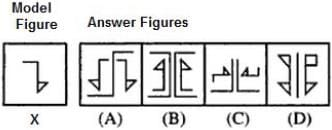Solution:
QUESTION: 4

Direction: In each of the following questions, you are given a figure (X) followed by four alternative figures (A), (B), (C) and (D) such that figure (X) is embedded in one of them.
Q. Find out the alternative figure which contains figure (X) as its part.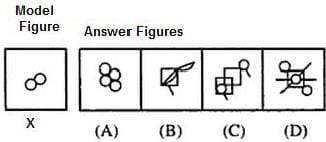Solution:
QUESTION: 5

Direction: In each of the following questions, you are given a figure (X) followed by four alternative figures (A), (B), (C) and (D) such that figure (X) is embedded in one of them.
Q. Find out the alternative figure which contains figure (X) as its part.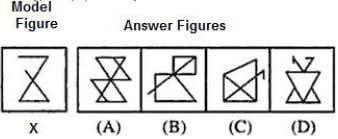Solution:
QUESTION: 6

Direction: In each of the following questions, you are given a figure (X) followed by four alternative figures (A), (B), (C) and (D) such that figure (X) is embedded in one of them.
Q. Find out the alternative figure which contains figure (X) as its part.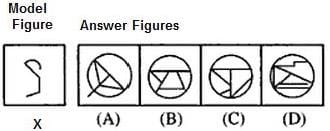Solution:
QUESTION: 7

Direction: In each of the following questions, you are given a figure (X) followed by four alternative figures (A), (B), (C) and (D) such that figure (X) is embedded in one of them.
Q. Find out the alternative figure which contains figure (X) as its part.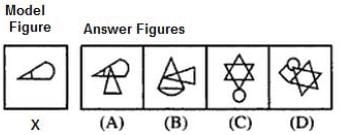Solution:
QUESTION: 8

Direction: In each of the following questions, you are given a figure (X) followed by four alternative figures (A), (B), (C) and (D) such that figure (X) is embedded in one of them.
Q. Find out the alternative figure which contains figure (X) as its part.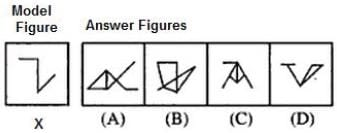Solution:
QUESTION: 9

Direction: In each of the following questions, you are given a figure (X) followed by four alternative figures (A), (B), (C) and (D) such that figure (X) is embedded in one of them.
Q. Find out the alternative figure which contains figure (X) as its part.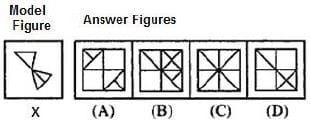Solution:
QUESTION: 10

Direction: In each of the following questions, you are given a figure (X) followed by four alternative figures (A), (B), (C) and (D) such that figure (X) is embedded in one of them.
Q. Find out the alternative figure which contains figure (X) as its part.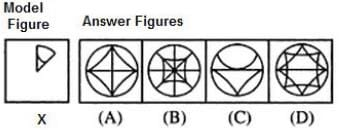Solution:Use Code STAYHOME200 and get INR 200 additional OFF Use Coupon Code